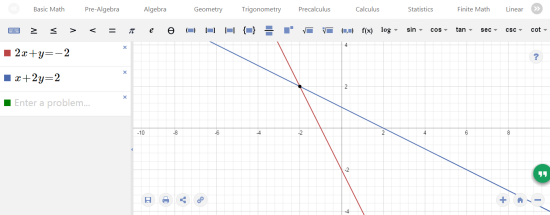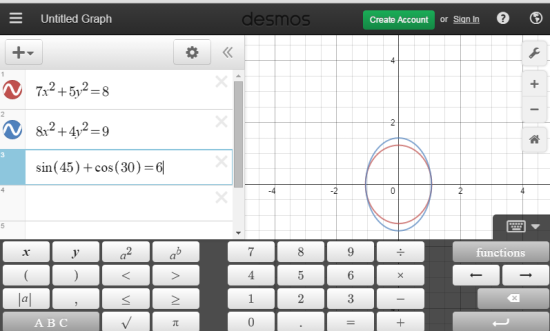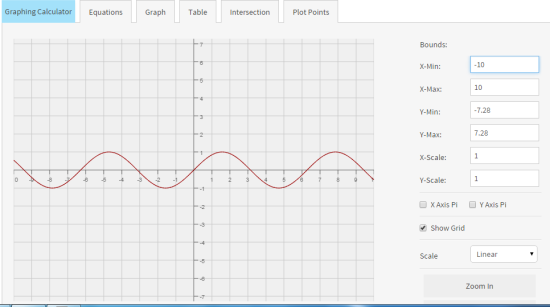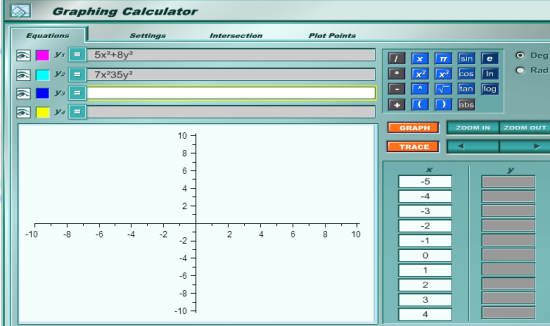Editor Ratings:
User Ratings:
[Total: 0 Average: 0]

Here’s a list of 5 free online graphing calculator websites. All of them are free to use and perform the desired task easily. You simply have to enter the equations and its values to get the corresponding graphical representation. These websites support various variables and functions for making the calculation and plotting of graph easy. You can also use them to solve complex problems within minutes.

The good thing about these online graphing calculators is that, you don’t have to worry about carrying a device wherever you go. Besides this, you are also saving some bucks for something else, like for your next date or for fuel. So, let’s have a look at each of them.

## 1. Mathway:Mathway is the most useful and best online graphing calculator among all included in this list. It lets you choose from multiple Mathematics concepts like Basic Math, Pre-Algebra, Algebra, Geometry, Trigonometry, Calculus, etc. The common symbols, numbers, etc. can be entered via physical keyboard while the special ones are shown to you on the screen. You have to click on them to use them. Mathway shows real time changes to the graph depending upon the changes made by you to the equations. You can easily save, share, and print the graph.

## 2. Desmos Graphing Calculator:Desmos Graphing Calculator is the second best online graphing calculator in the list. It features a virtual keyboard, text box for entering equations to solve, and the graph to see the resultant graphical presentation. The virtual keyboard includes commonly used mathematical symbols and signs. You can also use physical keyboard for inputting the problem(s). There is an option called Graph Settings which lets you configure the graph as per your requirement. The website also offers option to zoom-in and zoom-out of the graph.

There is also an option to add expressions, notes, folder, and images. The good thing is that if you choose to register with the website, then you can also save and share your graphs.

## 3. Meta-Calculator:Meta-Calculator is third website in the list. Its online graphing calculator UI has following elements: Equations, Graph, Table, Intersection, and Plot Points. You can enter up to 7 equations in one go and plot the graph with one click. There is a virtual keypad for entering specific mathematics symbols and functions. You can also switch between Radian and Degrees for getting the desired result.

On the graphing interface, you can choose to alter the values of X-min/max, Y-min/max, X-scale, and Y-scale. There is also an option to switch between scaling parameter: Linear, Semi-Log X, Semi-Log Y, and Log-log.

## 4. Graphing Calculator:The fourth website in the list Graphing Calculator has an interface similar to 90s games. There are following 4 options: Equations, Settings, Intersection, and Plot Points. You have to enter the equations in Equations option and Settings option lets you make changes to settings. On entering the equations, you can go for plotting the graph, finding the intersection points between two equations, and plot points. The website also lets you choose the color of line to represent each of the equation on the graph.

## 5. FooPlot:FooPlot is the last website in the list with minimalist interface. You can choose from Function, Polar, Parametric, and Points. On choosing the value type, enter the corresponding values to see the resulting graph. There are also various options which you can alter and adjust as per your requirement to get the desired output. FooPlot also has a Google Chrome extension.

These are the 5 free online graphing calculator websites for you to try out. Browse them to create graphs easily with nothing to carry with you all the time.

You may also like: 10 Free Websites to Print Graph Paper.

 Editor Ratings: User Ratings:[Total: 0 Average: 0] Free/Paid: Free